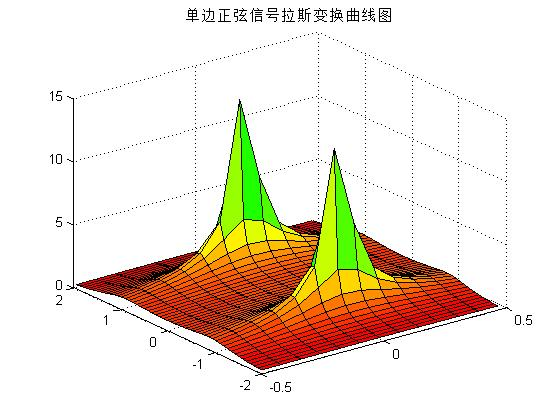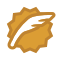# 单边正弦信号拉氏变换

拉普拉斯变换拉普拉斯变换应用数学中常用的一种积分变换，又名拉氏转换，其符号为$\displaystyle\mathcal{L} \left\{f(t)\right\}$。拉氏变换是一个线性变换，可将一个有引数实数tt ≥ 0）的函数转换为一个引数为复数s的函数。

## 基本定义

•$f(t)\,$是一个关于$t\,$函数，使得当$t<0\,$时候，$f(t)=0\,$
•$s\,$ 是一个复变量
•$\mathcal{L}$ 是一个运算符号，它代表对其对象进行拉普拉斯积分$\int_0^\infty e^{-st}\,dt$$F(s)\,$$f(t)\,$的拉普拉斯变换结果。$f(t)\,$拉普拉斯变换由下列式子给出：$F(s)\,=\mathcal{L}\left\{f(t)\right\}=\int_{0}^\infty f(t)\,e^{-st} \,dt$

## 拉普拉斯变换的基本性质$\mathcal{L}\left\{a f(t) + b g(t) \right\} = a \mathcal{L}\left\{ f(t) \right\} + b \mathcal{L}\left\{ g(t) \right\}$
• 时域微分（单边拉普拉斯变换）$\mathcal{L}\{f'\} = s \mathcal{L}\{f\} - f(0)$$\mathcal{L}\{f''\} = s^2 \mathcal{L}\{f\} - s f(0) - f'(0)$$\mathcal{L}\left\{ f^{(n)} \right\} = s^n \mathcal{L}\{f\} - s^{n - 1} f(0) - \cdots - f^{(n - 1)}(0)$
• s域微分$\mathcal{L}\{ t f(t)\} = -F'(s)$$\mathcal{L}\{\,t^nf(t)\} = (-1)^nD_s^n[F(s)]$
• s域积分$\mathcal{L}\left\{ \frac{f(t)}{t} \right\} = \int_s^\infty F(\sigma)\, d\sigma$$\mathcal{L} \left\{\frac{f(t)}{t^n}\right\} = \int_s^{\infty} \int_{\sigma_1}^{\infty} \cdots \int_{\sigma_{n-1}}^{\infty} F(\sigma_{n}) \, d\sigma_{n} \cdots \, d\sigma_2 \, d\sigma_1$$\mathcal{L}\left\{ \int_0^t f(\tau)\, d\tau \right\} = \mathcal{L}\left\{ 1 * f(t)\right\} = {1 \over s} \mathcal{L}\{f\}$$f(0^+)=\lim_{s\to \infty}{sF(s)}$ ，要求${F(s)}$为真分式，即分子的最高次小于分母的最高次，否则使用多项式除法${F(s)}$分解$f(\infty)=\lim_{s\to 0}{sF(s)}$　，要求${F(s)}$的所有极点都在左半复平面或原点为单极点。

•$s$域平移$\mathcal{L}\left\{ e^{at} f(t) \right\} = F(s - a)$$\mathcal{L}^{-1} \left\{ F(s - a) \right\} = e^{at} f(t)$
• 时域平移$\mathcal{L}\left\{ f(t - a) u(t - a) \right\} = e^{-as} F(s)$$\mathcal{L}^{-1} \left\{ e^{-as} F(s) \right\} = f(t - a) u(t - a)$$\mathcal{L} \left\{f(t) * g(t)\right\} = \frac{1}{2\pi i}\int_{c-i\infty}^{c+i\infty}F(\sigma)G(s-\sigma)\,d\sigma \ = \frac{1}{2\pi i} \mathcal{L}\{ f(t) \}*\mathcal{L}\{ g(t) \}$　，$c\,$是收敛区间的横坐标值，是一个实常数且大于所有$F(\sigma)\,$的个别点的实部值。$\mathcal{L}\left\{f(t) * g(t)\right\} = \frac{1}{2\pi i} \mathcal{L}\{ f(t) \}* \mathcal{L}\{ g(t) \}$

6-1：已知连续时间信号，求出该信号的拉普拉斯变换，并利用MATLAB绘制拉普拉斯变换的曲面图。

%绘制单边正弦信号拉普拉斯变换曲面图程序

注释： meshgrid() 用来产生 矩阵 s  s 是一个复频域 a 为实部 b为 虚部

ones()函数将器全部变成1

mesh 函数可以画出曲面图 三维的

abs() 取绝对值

close all;
a = -0.5:0.08:0.5;
b = -1.99:0.08:1.99;
[a,b] = meshgrid(a,b);
d = ones(size(a));
c = a +i*b;
c = c.*c;
c =c + d;
c = 1./c;
c = abs(c);
mesh(a,b,c);
surf(a,b,c);
axis([-0.5,0.5,-2,2,0,15]);
title('单边正弦信号拉斯变换曲线图');
colormap(hsv);wangxiaomingCSDN认证博客专家 架构 Spring Boot Redis

08-175003

#### 求单边余弦函数的傅里叶变换06-013万+

#### 两张张图让你明白时域， 频域和傅里叶变换

04-101090

#### 单边指数衰减信号的频谱

05-232万+

#### 信号处理——Hilbert变换及谱分析

10-133755

#### 利用希尔伯特变换进行的单边带幅度调制matlab程序

06-061301

#### MATLAB画信号单边频谱和双边频谱

04-282万+

#### matlab时频分析之连续小波变换cwt

12-187万+

#### 拉普拉斯变换的本质意义（好文！通俗易懂）

10-046286

#### 实信号和复信号频谱对比分析(双边带信号和单边带信号对比分析)©️2020 CSDN 皮肤主题: Age of Ai 设计师: meimeiellie点击重新获取扫码支付1.余额是钱包充值的虚拟货币，按照1:1的比例进行支付金额的抵扣。
2.余额无法直接购买下载，可以购买VIP、C币套餐、付费专栏及课程。余额充值